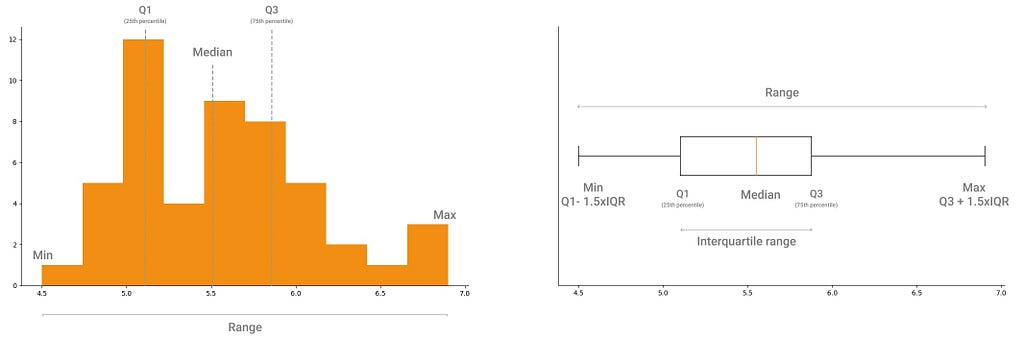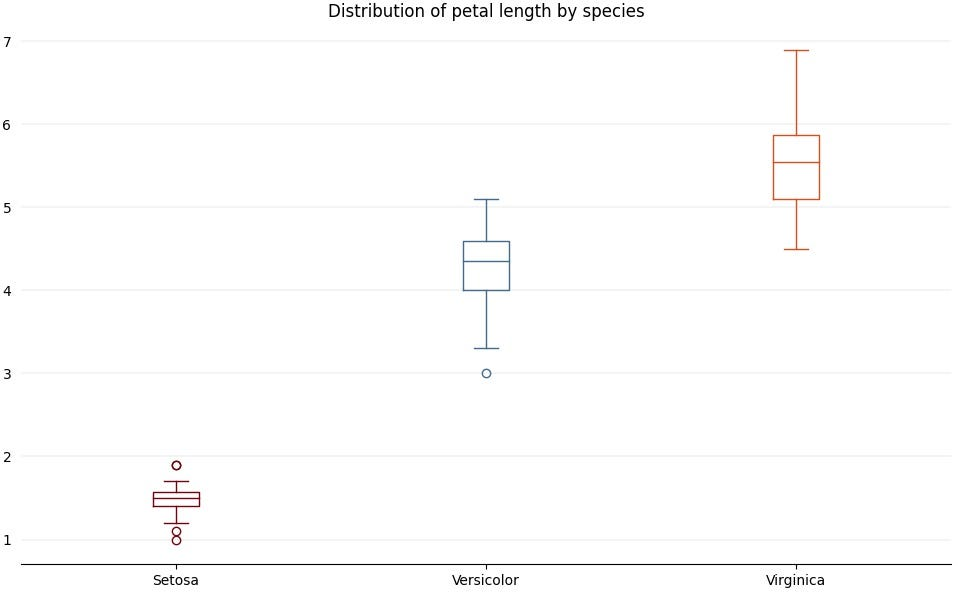Boxplots are underrated. They are jam-packed with insights about the underlying distribution, because they condense lots of information about your data into a small visualization.

• Understand the spread of the data.
• Spot outliers.
• Compare distributions, and how small tweaks in the boxplot visualization make it easier spot differences between distributions.

### Understanding the spread of the data

During exploratory data analysis, boxplots can be a great complement to histograms.

With histograms it’s easy to see the shape and trends in a distribution. Because histograms highlight how frequently each data point occurs in the distribution.

Boxplots don’t focus directly on frequency, but instead on the range of values in the distribution.

We are used to think in terms of frequency and comparing proportions. That’s why we’re so comfortable interpreting the insights of an histogram, where we can spot the values where most data is concentrated around, and we can see the shape of the distribution.

With a boxplot, we can extract the same insights as with an histogram. And while we can visualize the shape of the distribution with an histogram, a boxplot highlights the summary metrics that give the distribution its shape. The summary metrics we can extract from a boxplot are:

• Quantiles, specifically the first and third quantiles, which correspond to the 25th and 75th percentiles.
• Median, the mid-point in the distribution, which also corresponds to the 50th percentile.
• Interquartile range (IQR), the width between the third and first quantiles. Expressed mathematically, we have IQR = Q3 — Q1.
• Min, minimum value in the dataset excluding outliers, which corresponds to Q1–1.5xIQR.
• Max, maximum value in the dataset, excluding outliers, which corresponds to Q3+ 1.5xIQR.Summary metrics you can extract from an histogram and a boxplot.

### Spot outliers

Visualized in a boxplot outliers typically show up as circles. But as you’ll see in the next section, you can customize how outliers are represented 😀

If your dataset has outliers, it will be easy to spot them with a boxplot. There are different methods to determine that a data point is an outlier. The most widely known is the 1.5xIQR rule.

### 1.5xIQR rule

Outliers are extreme observations in the dataset. So a rule of thumb to determine if a data point is extreme is to compare it against the interquartile range.

It makes sense to use the interquartile range (IQR) to spot outliers. The IQR is the range of values between the first and third quartiles, i.e., 25th and 75th percentiles, so it will include the majority of the data points in the dataset.

But why 1.5 times the interquartile range? This is related to an important characteristic of the Normal Distribution known as the 68–95–99 rule.

With the 68–95–99 rule, we know that:

• 68% of the data is within one standard deviation above or below the mean,
• 95% of the data is within two standard deviations from the mean,
• 99.7% of the data is within three standard deviations from the mean.

Only very few data points will be beyond three standard deviations from the mean, more precisely, only 0.3% of the data points. So any data point that is seen farther than three standard deviations is considered extreme.

To check if a data point is an outlier and check if it falls farther than three standard deviations, we calculate:

• Q1–1.5xIQR,
• Q3 + 1.5xIQR.

These represent the lower and upper bounds of the area in the distribution that is not considered extreme. Which ends up being approximately 3 standard deviations from the mean.

The multiplying factor is 1.5, because any number greater than that would result in a range bigger than 3 standard deviations. So, mathematicians settled in a number in the middle.

Any data point lower than the lower bound or greater than the upper bound is an outlier:

• (data point value) < Q1–1.5xIQR, then it’s an outlier.
• (data point value) > Q3 + 1.5xIQR, then it’s an outlier.

### Customizing boxplots to compare distributions

Boxplots are also a great tool to compare different distributions.

Let’s compare the distributions of petal length for flowers in the Iris dataset.

Here’s how you can create this plot.

`import numpy as npimport pandas as pdfrom sklearn import datasetsimport matplotlib.pyplot as plt`
`# Load Iris datasetiris = datasets.load_iris()`
`# Preparing Iris datasetiris_data = pd.DataFrame(data=iris.data, columns=['sepal_length', 'sepal_width', 'petal_length', 'petal_width'])`
`iris_target = pd.DataFrame(data=iris.target, columns=['species'])iris_df = pd.concat([iris_data, iris_target], axis=1)`
`# Add species nameiris_df['species_name'] = np.where(iris_df['species'] == 0, 'Setosa', None)`
`iris_df['species_name'] = np.where(iris_df['species'] == 1, 'Versicolor', iris_df['species_name'])`
`iris_df['species_name'] = np.where(iris_df['species'] == 2, 'Virginica', iris_df['species_name'])`
`# Prepare petal length by species datasetssetosa_petal_length = iris_df[iris_df['species_name'] == 'Setosa']['petal_length']`
`versicolor_petal_length = iris_df[iris_df['species_name'] == 'Versicolor']['petal_length']`
`virginica_petal_length = iris_df[iris_df['species_name'] == 'Virginica']['petal_length']`
`# Visualize petal length distribution for all species`
`fig, ax = plt.subplots(figsize=(12, 7))`
`# Remove top and right borderax.spines['top'].set_visible(False)ax.spines['right'].set_visible(False)ax.spines['left'].set_visible(False)`
`# Remove y-axis tick marksax.yaxis.set_ticks_position('none')`
`# Add major gridlines in the y-axisax.grid(color='grey', axis='y', linestyle='-', linewidth=0.25, alpha=0.5)`
`# Set plot titleax.set_title('Distribution of petal length by species')`
`# Set species names as labels for the boxplotdataset = [setosa_petal_length, versicolor_petal_length, virginica_petal_length]`
`labels = iris_df['species_name'].unique()ax.boxplot(dataset, labels=labels)`
`plt.show()`

We can extract a few insights from this plot:

• Iris Setosa has a much smaller petal length than Iris Versicolor and Virginica. It ranges from approximately 1 to 2 centimeters.
• The range of petal length of Iris Virginica is bigger than both the ranges of values for Iris Setosa and Versicolor. We can see that from how tall the box is for Iris Virginica compared to the other two.
• Both Iris Setosa and Veriscolor have outliers.

We can also confirm these insights by looking at the summary metrics of each distribution.

And here’s how you can compute these metrics.

`def get_summary_statistics(dataset):        mean = np.round(np.mean(dataset), 2)    median = np.round(np.median(dataset), 2)    min_value = np.round(dataset.min(), 2)    max_value = np.round(dataset.max(), 2)`
`    quartile_1 = np.round(dataset.quantile(0.25), 2)    quartile_3 = np.round(dataset.quantile(0.75), 2)`
`    # Interquartile range    iqr = np.round(quartile_3 - quartile_1, 2)`
`    print('Min: %s' % min_value)    print('Mean: %s' % mean)    print('Max: %s' % max_value)    print('25th percentile: %s' % quartile_1)    print('Median: %s' % median)    print('75th percentile: %s' % quartile_3)    print('Interquartile range (IQR): %s' % iqr)    print('Setosa summary statistics')`
`print('\n\nSetosa summary statistics')get_summary_statistics(setosa_petal_length)`
`print('\n\nVersicolor summary statistics')get_summary_statistics(versicolor_petal_length)`
`print('\n\nVirginica summary statistics')get_summary_statistics(virginica_petal_length)`

#### Customizing your boxplot

At first glance, it’s hard to distinguish between the boxplots of the different species. The labels at the bottom are the only visual clue that we’re comparing distributions.

We can use the properties of the boxplot to customize each box. Since properties are applies to all the data that is given to the boxplot method, we can’t take the approach of the last plot and use an array with the petal length for each species as an input.

We’ll have to plot the petal length for each species and applies properties to each one of them.

We’re going to use the following parameters:

• positions: position of the boxplot in the plot area. We don’t want to plot each species’ boxplot on top of each other, so we use this to set the position in the x-axis where each boxplot will be drawn.
• medianprops: dictionary of properties applied to median line inside the boxplot.
• whiskerprops: dictionary of properties applied to the whiskers.
• capprops: dictionary of properties applied to the caps on the whiskers.
• flierprops: dictionary of properties applied to outliers.

There are other several properties we can customize. In this example I’m going to just add a different color for each of the boxplots, so it’s easier to see that we’re visualizing different distributions.Comparing petal length for the Iris dataset, with custom colors for each species.
`fig, ax = plt.subplots(figsize=(12, 7))`
`# Remove top and right borderax.spines['top'].set_visible(False)ax.spines['right'].set_visible(False)ax.spines['left'].set_visible(False)`
`# Remove y-axis tick marksax.yaxis.set_ticks_position('none')`
`# Set plot titleax.set_title('Distribution of petal length by species')`
`# Add major gridlines in the y-axisax.grid(color='grey', axis='y', linestyle='-', linewidth=0.25, alpha=0.5)`
`# Set species names as labels for the boxplotdataset = [setosa_petal_length, versicolor_petal_length, virginica_petal_length]labels = iris_df['species_name'].unique()`
`# Set the colors for each distributioncolors = ['#73020C', '#426A8C', '#D94D1A']colors_setosa = dict(color=colors)colors_versicolor = dict(color=colors)colors_virginica = dict(color=colors)`
`# We want to apply different properties to each species, so we're going to plot one boxplot# for each species and set their properties individually# positions: position of the boxplot in the plot area# medianprops: dictionary of properties applied to median line# whiskerprops: dictionary of properties applied to the whiskers# capprops: dictionary of properties applied to the caps on the whiskers# flierprops: dictionary of properties applied to outliers`
`ax.boxplot(dataset, positions=, labels=[labels], boxprops=colors_setosa, medianprops=colors_setosa, whiskerprops=colors_setosa, capprops=colors_setosa, flierprops=dict(markeredgecolor=colors))`
`ax.boxplot(dataset, positions=, labels=[labels], boxprops=colors_versicolor, medianprops=colors_versicolor, whiskerprops=colors_versicolor, capprops=colors_versicolor, flierprops=dict(markeredgecolor=colors))`
`ax.boxplot(dataset, positions=, labels=[labels], boxprops=colors_virginica, medianprops=colors_virginica, whiskerprops=colors_virginica, capprops=colors_virginica, flierprops=dict(markeredgecolor=colors))`
`plt.show()`

That’s it! You can use boxplots to explore your data and customize your visualizations so it’s easier to extract insights.